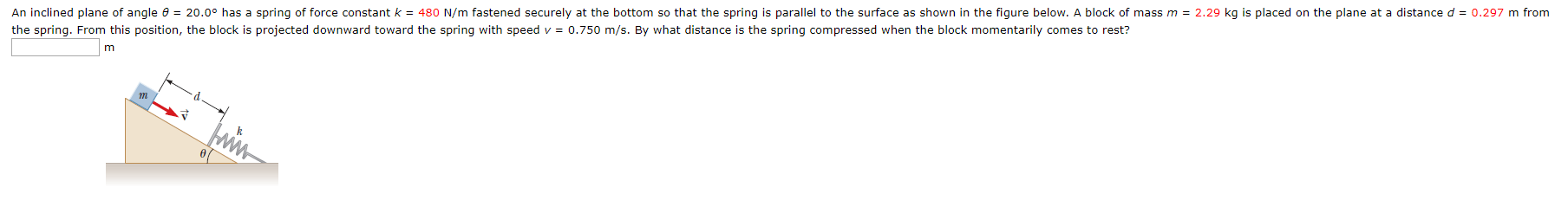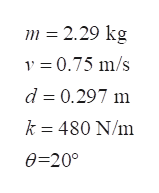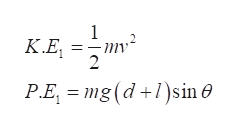20.0° has a spring of force constant k = 480 N/m fastened securely at the bottom so that the spring is parallel to the surface as shown in the figure below. A block of mass m 2.29 kg is placed on the plane at a distance d = 0.297 m fromAn inclined plane of angle ethe spring. From this position, the block is projected downward toward the spring with speed v0.750 m/s. By what distance is the spring compressed when the block momentarily comes to rest?

Questionhelp_outlineImage Transcriptionclose20.0° has a spring of force constant k = 480 N/m fastened securely at the bottom so that the spring is parallel to the surface as shown in the figure below. A block of mass m 2.29 kg is placed on the plane at a distance d = 0.297 m from An inclined plane of angle e the spring. From this position, the block is projected downward toward the spring with speed v 0.750 m/s. By what distance is the spring compressed when the block momentarily comes to rest? fullscreen
Step 1

Let m be the mass of the block, d be the distance between the uncompressed spring and block, v be the velocity of the block, l be the length of the uncompressed spring, x be the distance by which the spring is compressed, k be the spring constant.

Write the given numerical values.help_outlineImage Transcriptionclosem 2.29 kg v 0.75 m/s d 0.297 m k 480 N/m 0-20° fullscreen
Step 2

Refer the following diagram to analyse the motion of the block and compression of spring.

Step 3

Write the expression for initial kinetic and potential energy of the system. As t...help_outlineImage Transcriptionclose1 K.E 2 P.E, mg (d)sin sin0 + / fullscreen

Want to see the full answer?

See Solution

Want to see this answer and more?

Our solutions are written by experts, many with advanced degrees, and available 24/7

See Solution
Tagged in

Work,Power and Energy